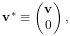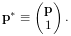# 2.3 Points and Vectors

The rotations and transforms described above can be used to transform both vectors and points in space.

Vectors are most commonly implemented using Vector3d, while points can be implemented using the subclass Point3d. The only difference between Vector3d and Point3d is that the former ignores the translational component of rigid and affine transforms; i.e., as described in Sections A.2 and A.3, a vector v has an implied homogeneous representation of(2.1)

while the representation for a point p is(2.2)

Both classes provide a number of methods for applying rotational and affine transforms. Those used for rotations are

void transform (R);             // this = R * this
void transform (R, v1);         // this = R * v1
void inverseTransform (R);      // this = inverse(R) * this
void inverseTransform (R, v1);  // this = inverse(R) * v1

where R is a rotation matrix and v1 is a vector (or a point in the case of Point3d).

The methods for applying rigid or affine transforms include:

void transform (X);             // transforms this by X
void transform (X, v1);         // sets this to v1 transformed by X
void inverseTransform (X);      // transforms this by the inverse of X
void inverseTransform (X, v1);  // sets this to v1 transformed by inverse of X

where X is a rigid or affine transform. As described above, in the case of Vector3d, these methods ignore the translational part of the transform and apply only the matrix component (R for a RigidTransform3d and A for an AffineTransform3d). In particular, that means that for a RigidTransform3d given by X and a Vector3d given by v, the method calls

v.transform (X.R)
v.transform (X)

produce the same result.